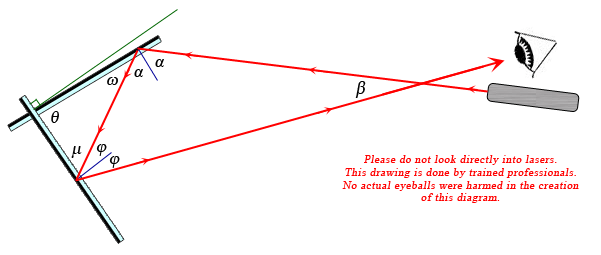SEARCH HOMEMath Central Quandaries & QueriesQuestion from Peter, a student: The reflecting surfaces of two intersecting flat mirrors are at an angle θ (0° < θ < 90°). For a light ray that strikes the horizontal mirror, show that the emerging ray will intersect the incident ray at an angle β = 180°  2θ.Hi Peter.

I drew a diagram and labelled those and other angles with variables from the Greek alphabet, using rules of reflection to indicate which angles have the same measure as other angles:Note that the "angle of incidence" equals the "angle of reflection" as measured from the perpendicular to the mirror surfaces.

The sum of the angles of the red triangle is 180 degrees:

2α + 2φ + β = 180.

Also, we see that ω and α are complementary angles, so α = 90 - ω. For the same reason, φ= 90 - μ.

When we substitute this into the first equation, we get:

2(90 - ω) + 2(90 - μ) + β = 180.

We also know that the other triangle's angles add to 180 degrees: θ + μ + ω = 180. So ω = 180 - μ - θ.

Substituting this into the previous equation gives:

2(90 - (180 - μ - θ)) + 2(90 - μ) + β = 180.

Solve for β.

Cheers,
Stephen La Rocque.Math Central is supported by the University of Regina and The Pacific Institute for the Mathematical Sciences.